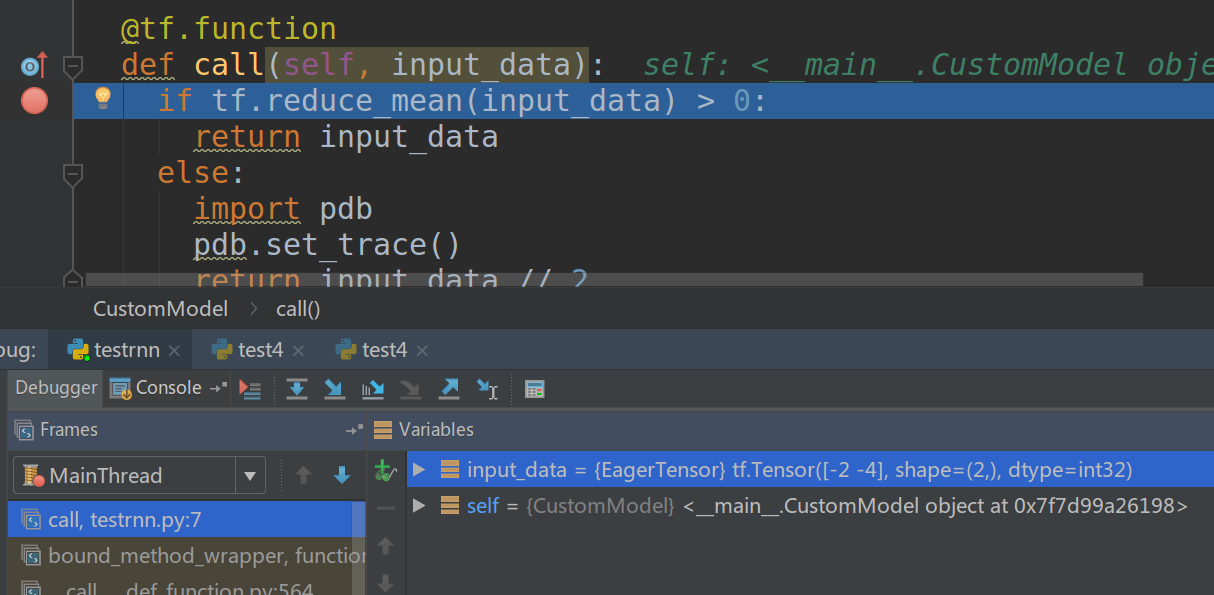# TensorFlow 2.X教程(二)：TensorFlow 2.X和1.X的比较

Posted by lili on September 15, 2020

## TensorFlow 2.X的改进

TensorFlow 2.X的很多改进都让用户更加高效，比如移除冗余API、让API更加一致(统一的RNN API统一的Optimizer API)和Eager execution。下面我们来看一下主要的改动。

### Eager execution

TensorFlow 1.X需要用户使用tf.*里的API手动构建计算图。然后用session.run()传入输入tensor并且计算某些输出tensor。TensorFlow 2.X默认是Eager执行模式，我们在定义一个Operation的时候会动态构造计算图并且马上计算。这样的好处就是我们的代码就像在执行普通的Python代码，Graph和Session等实现细节概念都被隐藏在后面了。

Eager执行的另外一个好处就是不再需要tf.control_dependencies了(如果不知道也没有关系，以后不会再用到了)，因为Tensorflow的计算图是按照Python代码的顺序执行。

### 无全局变量

TensorFlow 1.X严重依赖隐式的全局命名空间。当我们调用tf.Variable()创建一个变量时，它会放到一个默认的Graph里，即使对应的Python变量超出了它的生命周期(被垃圾回收)了，这个变量依然在Graph里。你可以从图中恢复出这个变量，但是你必须得知道它的名字。这通常很困难，因为很多变量并不是你手动创建的，而是诸如tf.layers.conv2d之类的API帮你创建的，你并不知道它是怎么命名变量的(除非去看源代码)。为了解决这个问题搞出了很多复杂的东西：variable_scope，全局的集合，以及tf.get_global_step()和tf.global_variables_initializer()等工具类函数，optimzier隐式的对所有”可训练”的变量计算梯度，等等。TensorFlow 2.X把这些都抛弃掉了，你只需要跟踪(track)你的Python变量就可以了，如果你不再引用你的tf.Variable，它就会被回收，对应的计算图里就不会再有它。

### 使用函数替代Session

session.run()很像函数调用：你通过feed dict传入输入，调用后就会得到输出。不过它和普通函数还是很不相同，普通函数的输入和输出是提前定义好的，而session.run可以在调用的时候指定输入和输出，当然输入输出只能是计算图中提前定义好的。在TensorFlow 2.X里，我们可以用tf.function来装饰(decorate)某个普通函数，则TensorFlow会使用JIT把这个普通的Python函数编译成一个计算图。编译后可以获得原来计算图的所有好处，包括：

• 性能
• 就像编译器和解释器的区别，编译后的代码运行效率更高，编译时可以做很多性能优化
• 可移植性
• 函数(计算图)可以导入或者导出，这样即使没有原来的(Python)代码，依然可以使用它。

# TensorFlow 1.X
outputs = session.run(f(placeholder), feed_dict={placeholder: input})
# TensorFlow 2.0
outputs = f(input)


    for/while -> tf.while_loop (支持break和continue)
if -> tf.cond
for _ in dataset -> dataset.reduce


AutoGraph支持任意嵌套的控制流，这让它非常适合实现很复杂的模型，比如序列模型、强化学习和自定义循环等等。

## TensorFlow 2.X推荐用法

### 使用Keras的Layer或者Model来管理变量

Keras的Layer或者Model会用variables和trainable_variables这些属性来自己管理变量，如果它的成员变量有Layer或者Model，它也会递归的管理它们的变量。比如自己管理变量：

def dense(x, W, b):
return tf.nn.sigmoid(tf.matmul(x, W) + b)

@tf.function
def multilayer_perceptron(x, w0, b0, w1, b1, w2, b2 ...):
x = dense(x, w0, b0)
x = dense(x, w1, b1)
x = dense(x, w2, b2)



# 每个Layer都是callable
layers = [tf.keras.layers.Dense(hidden_size, activation=tf.nn.sigmoid) for _ in range(n)]
perceptron = tf.keras.Sequential(layers)

# layers.trainable_variables => returns [w3, b3]
# perceptron.trainable_variables => returns [w0, b0, ...]



Keras的Layer或者Model继承了tf.train.Checkpointable并且能够和@tf.function集成，这可以方便的对变量进行checkpoint或者用SavedModel保存整个模型的计算图。即使我们不用Keras的fit()这样的API也可以使用它来帮我们管理变量和进行tf.function的集成。

trunk = tf.keras.Sequential([...])

# 在主数据集上训练
for x, y in main_dataset:
prediction = path1(x, training=True)

# 使用第二个数据集进行fine-tune，重用主干网络
for x, y in small_dataset:
prediction = path2(x, training=True)

# 保存模型
tf.saved_model.save(trunk, output_path)



### 组合使用tf.data.Datasets和@tf.function

@tf.function
def train(model, dataset, optimizer):
for x, y in dataset:
prediction = model(x, training=True)
loss = loss_fn(prediction, y)


model.compile(optimizer=optimizer, loss=loss_fn)
model.fit(dataset)


### Python的控制流会利用AutoGraph

AutoGraph会把Python的if-else和while等依赖于数据的条件/循环语句编译成Graph的诸如tf.cond或者tf.while_loop等等价操作。

class DynamicRNN(tf.keras.Model):

def __init__(self, rnn_cell):
super(DynamicRNN, self).__init__(self)
self.cell = rnn_cell

def call(self, input_data):
# [batch, time, features] -> [time, batch, features]
input_data = tf.transpose(input_data, [1, 0, 2])
outputs = tf.TensorArray(tf.float32, input_data.shape)
state = self.cell.zero_state(input_data.shape, dtype=tf.float32)
for i in tf.range(input_data.shape):
output, state = self.cell(input_data[i], state)
outputs = outputs.write(i, output)
return tf.transpose(outputs.stack(), [1, 0, 2]), state


rnn_cell = tf.compat.v1.nn.rnn_cell.BasicRNNCell(4)
rnn = DynamicRNN(rnn_cell)

inputs = np.random.random([8, 10, 12]).astype(np.float32)
outputs, last_hidden = rnn(inputs)
print(outputs.shape)   -> (8, 10, 4)
print(last_hidden.shape)  -> (8, 4)


### 使用tf.metrics和tf.summary

summary_writer = tf.summary.create_file_writer('/tmp/summaries')
with summary_writer.as_default():
tf.summary.scalar('loss', 0.1, step=42)


def train(model, optimizer, dataset, log_freq=10):
avg_loss = tf.keras.metrics.Mean(name='loss', dtype=tf.float32)
for images, labels in dataset:
loss = train_step(model, optimizer, images, labels)
avg_loss.update_state(loss)
if tf.equal(optimizer.iterations % log_freq, 0):
tf.summary.scalar('loss', avg_loss.result(), step=optimizer.iterations)
avg_loss.reset_states()

def test(model, test_x, test_y, step_num):
loss = loss_fn(model(test_x, training=False), test_y)
tf.summary.scalar('loss', loss, step=step_num)

train_summary_writer = tf.summary.create_file_writer('/tmp/summaries/train')
test_summary_writer = tf.summary.create_file_writer('/tmp/summaries/test')

with train_summary_writer.as_default():
train(model, optimizer, dataset)

with test_summary_writer.as_default():
test(model, test_x, test_y, optimizer.iterations)



with train_summary_writer.as_default():
train(model, optimizer, dataset)


tensorboard --logdir /tmp/summaries


### 使用tf.config.experimental_run_functions_eagerly()进行调试

@tf.function
def f(x):
if x > 0:
import pdb
pdb.set_trace()
x = x + 1
return x

tf.config.experimental_run_functions_eagerly(True)
f(tf.constant(1))图：tf.function的调试

class CustomModel(tf.keras.models.Model):

@tf.function
def call(self, input_data):
if tf.reduce_mean(input_data) > 0:
return input_data
else:
import pdb
pdb.set_trace()
return input_data // 2

tf.config.experimental_run_functions_eagerly(True)
model = CustomModel()
model(tf.constant([-2, -4]))图：tf.keras的调试